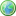Accessibility options:

# Pythagoras' theorem and applications to basic problems resources

Show me all resources applicable to

### Video with captions which require edits (2)Pythagoras' theorem
Pythagoras theorem - the square on the hypotenuse is equal to the sum of the squares on the other two sides - is well known. In this tutorial we revise the theorem and use it to solve problems in right-angled triangles. A less familiar form of the theorem is also considered. (Mathtutor Video Tutorial) The video is released under a Creative Commons license Attribution-Non-Commercial-No Derivative Works and the copyright is held by Skillbank Solutions Ltd.Pythagoras' Theorem - an Animation
This mathtutor animation shows visually that the square on the hypotenuse is equal to the sum of the squares on the other two sides. The video is released under a Creative Commons license Attribution-Non-Commercial-No Derivative Works and the copyright is held by Skillbank Solutions Ltd.

Website design by Pink Mayhem, Leicester